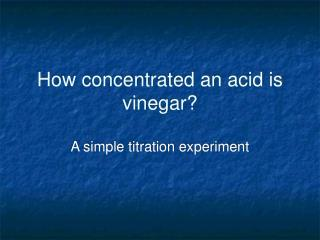# How concentrated an acid is vinegar? - PowerPoint PPT PresentationDownload PresentationHow concentrated an acid is vinegar?

Presentation Description
Download Presentation## How concentrated an acid is vinegar?

- - - - - - - - - - - - - - - - - - - - - - - - - - - E N D - - - - - - - - - - - - - - - - - - - - - - - - - - -
##### Presentation Transcript

1. How concentrated an acid is vinegar? A simple titration experiment

2. The big idea • Acids and bases neutralize each other • A base of known concentration can be used to determine the concentration of an unknown acid • At the equivalence point, the moles of acid and base molecules are exactly equal • Color change is the indication of equivalence

3. The reaction • Acids and bases neutralize each other • A base of known concentration can be used to determine the concentration of an unknown acid • At the equivalence point, the moles of acid and base molecules are exactly equal • Color change is the indication of equivalence

4. Each mole of NaOH neutralizes one mole of acetic acid • For a 1M solution, each mL is 0.001 moles of NaOH

5. When you add EXACTLY equal moles of NaOH, the phenopthalein will turn pink and STAY pink • the critical ONE DROP is the one that does it

6. Calibrate the dropper: How many mL in one drop? • Count the drops to fill a 4 mL cuvette

7. Do the calculation

8. Results • What was your measured concentration of acetic acid in the vinegar? • How does this compare with what the bottle says?